# Procedure for the simulation experiment of four Fourier analysis method

Exp-3(a) Relationship between CTFS and CTFT of periodic signal

Exp-3(b) Relationship between CTFS and CTFT of aperiodic signal

Exp-3(c) Relationship between DTFS and DTFT of periodic signal

Exp-3(d) Relationship between DTFS and DTFT of aperiodic signal

Exp-3(e) Relationship between CTFT and DTFT

Exp-3(f) Relation between four Fourier methods

Exp-3(a) Relationship between CTFS and CTFT of periodic signal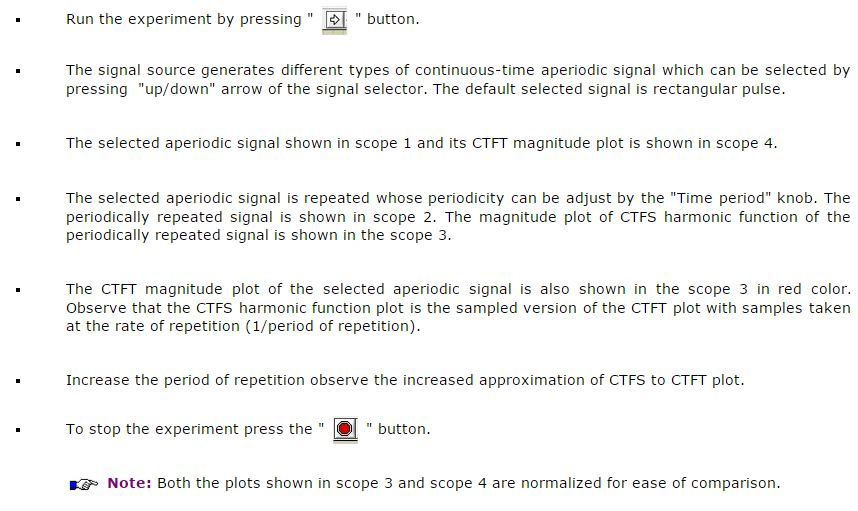Exp-3(c) Relationship between DTFS and DTFT of periodic signal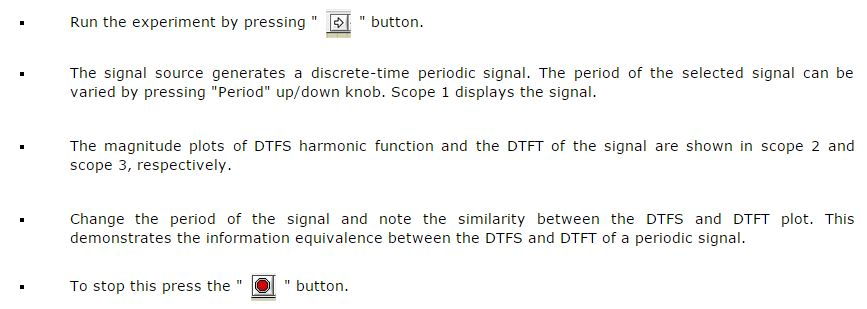Exp-3(d) Relationship between DTFS and DTFT of aperiodic signal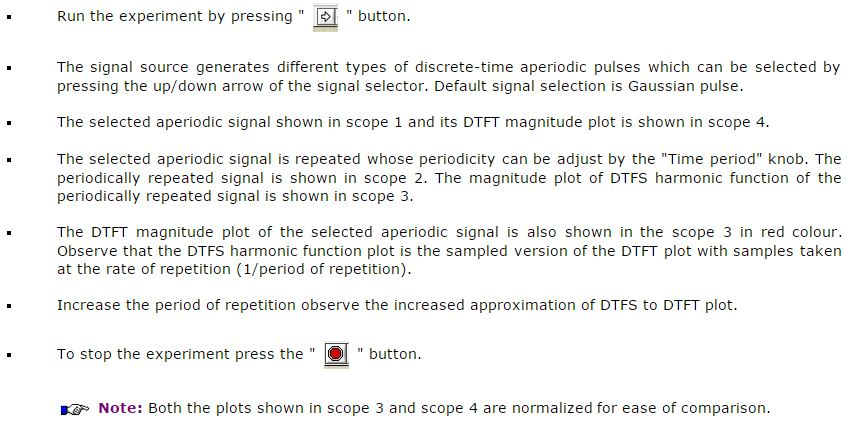Exp-3(e) Relationship between CTFT and DTFT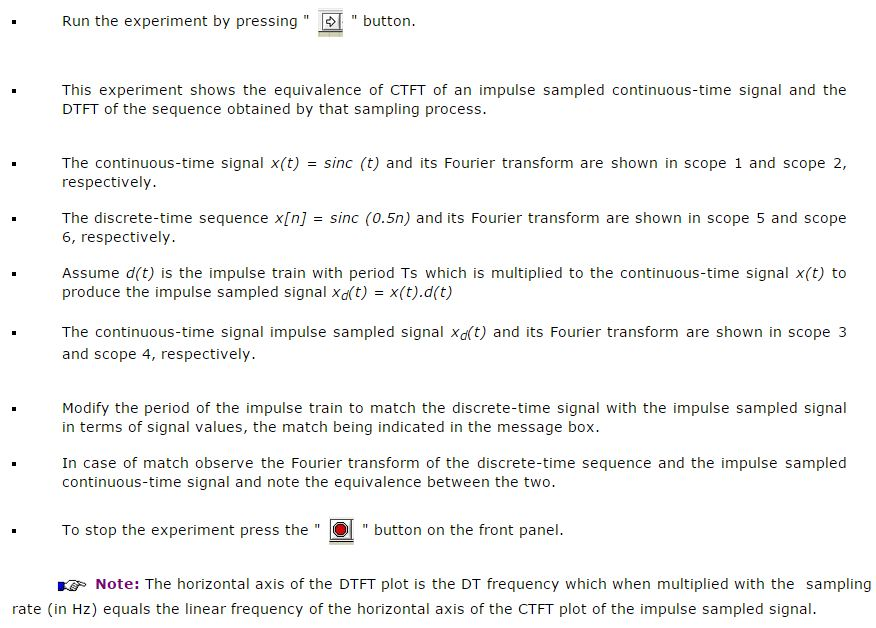Exp-3(f) Relationship between four Fourier methods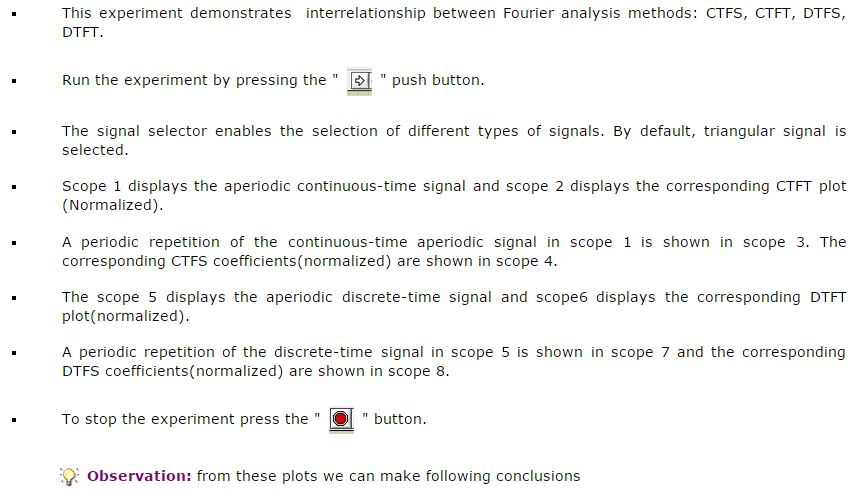1. In case of periodic signals (both continuous-time and discrete-time) the corresponding Fourier spectra are discrete.

2. In case of discrete signals (both continuous-time and discrete-time) the corresponding Fourier spectra are repetitive.

3. From observations (1) and (2) we note that "If a signal is periodic in one domain then its Fourier transform would be discrete in other domain".